Function Repository Resource:

# LinearCombination

Decompose a vector into a linear combination of a set of vectors

Contributed by: Wolfram|Alpha Math Team
 ResourceFunction["LinearCombination"][{u},{vi}] returns a list of coefficients that express u as a linear combination of the basis vectors vi. ResourceFunction["LinearCombination"][{u}, {vi}, type] returns the given representation type that express u as a linear combination of the vi.

## Details and Options

The input type can be "Association", "Coefficients" or "FullDecomposition" and defaults to "Coefficients".
The basis set need not be linearly independent.

## Examples

### Basic Examples (1)

Express a vector as a combination of two vectors:

 In:=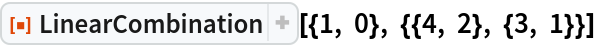Out=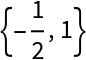### Scope (3)

Express the output as an Association:

 In:=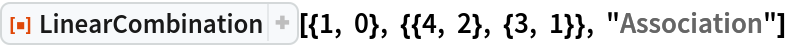Out=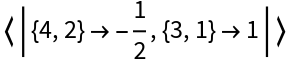Display the linear combination as an Inactive expression:

 In:=Out=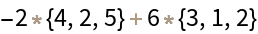Verify the decomposition is correct:

 In:=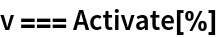Out=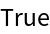Express a matrix in terms of a set of matrices:

 In:=Out=Verify the decomposition is correct:

 In:=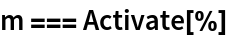Out=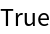### Possible Issues (2)

If no linear combination is found, LinearCombination returns unevaluated:

 In:=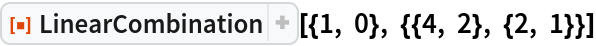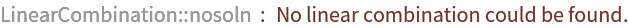Out=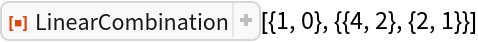If the basis set is not linearly independent, LinearCombination will return one combination from the infinite set of possibilities:

 In:=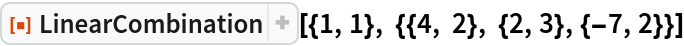Out=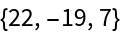## Publisher

Wolfram|Alpha Math Team

## Version History

• 2.0.0 – 23 March 2023
• 1.0.0 – 01 April 2020